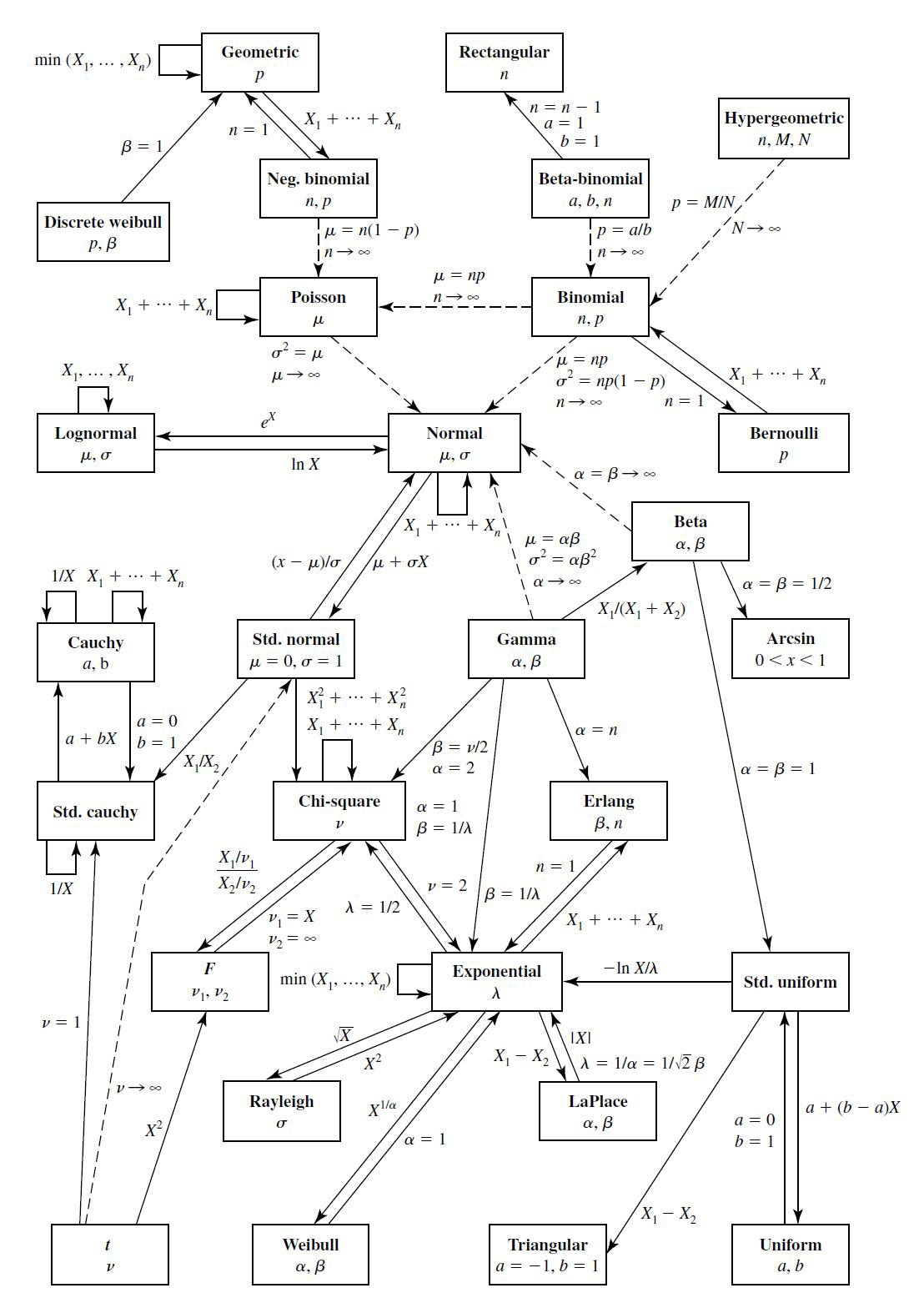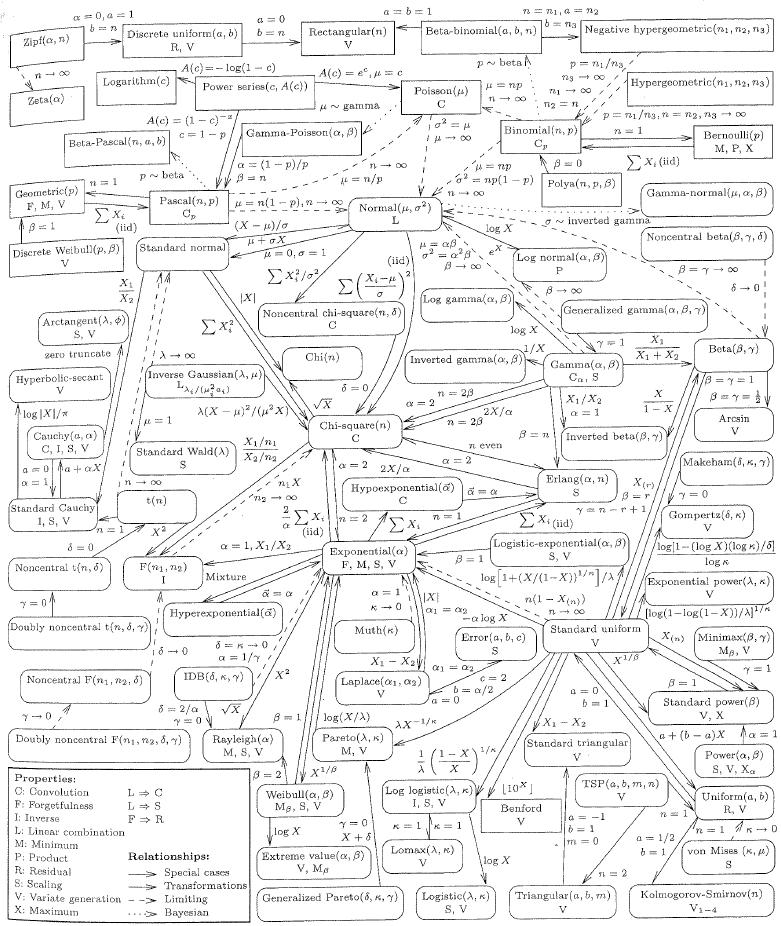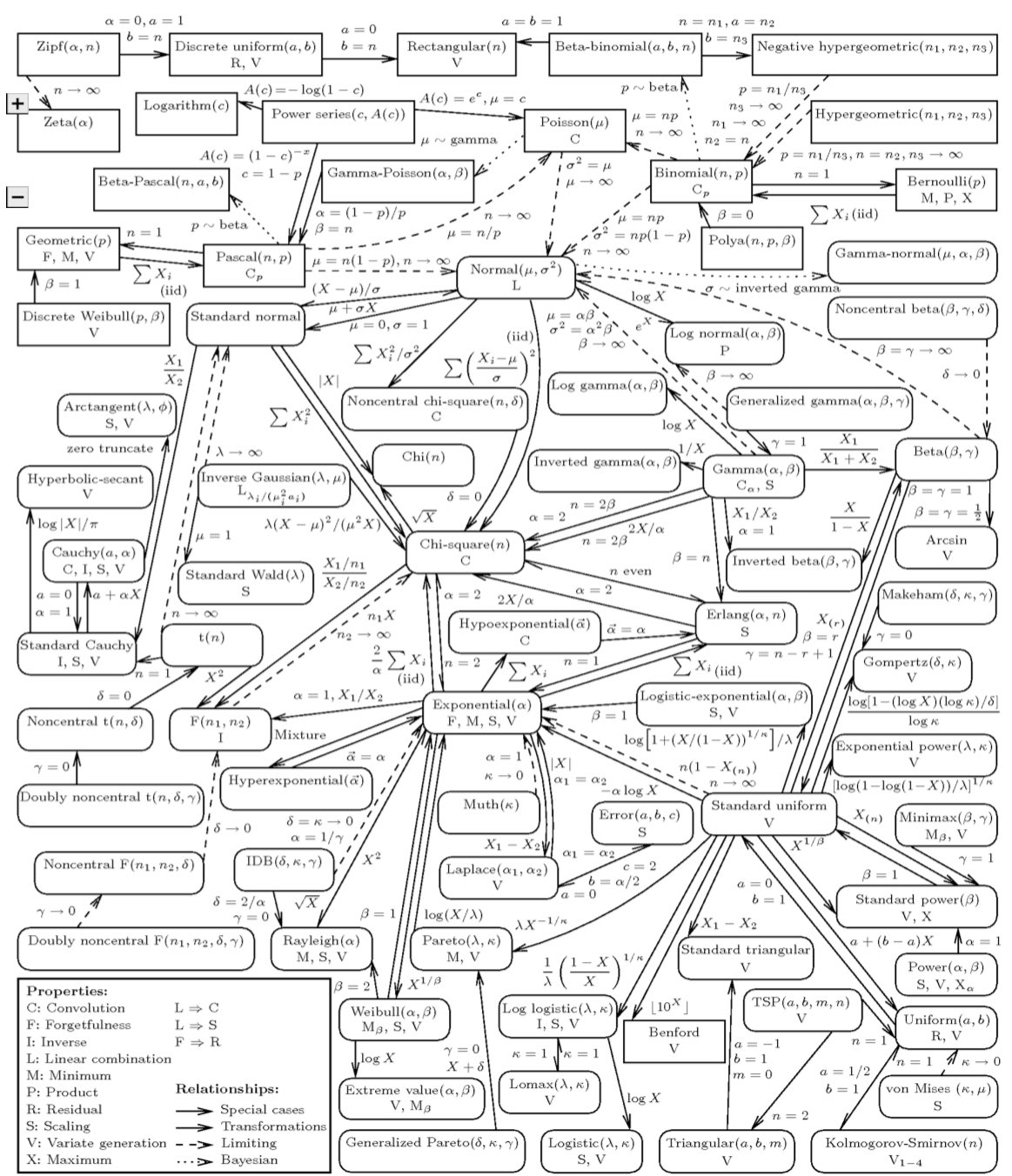If you find any of this useful, please consider donating via PayPal to help keep this site going.# Probability Distribution Web

1/31/17

Have you ever wondered how to get from a normal distribution ("bell curve") to a uniform distribution ("flat curve")? If so, this article was meant for you!

Probability distributions are often related to each other. Sometimes you can apply a transformation, or take a limit, or make a ratio, or let some parameters equal a certain value to move from one probability distribution to another. For example, probably the most well known to students is, if you take a Z transformation, Z = (x-mu)/sigma, you move from a normal distribution with mean mu and standard deviation sigma to a standard normal distribution with mean 0 and standard deviation 1.

I've put two very useful "webs" below. The first ones are a small web from the great book Statistical Inference by Casella and Berger. The second ones are a more detailed web from The American Statistician, February 2008, Vol 62, No 1. I've also linked to the .pdf versions of these files here and here.Please let me know if you find these useful.